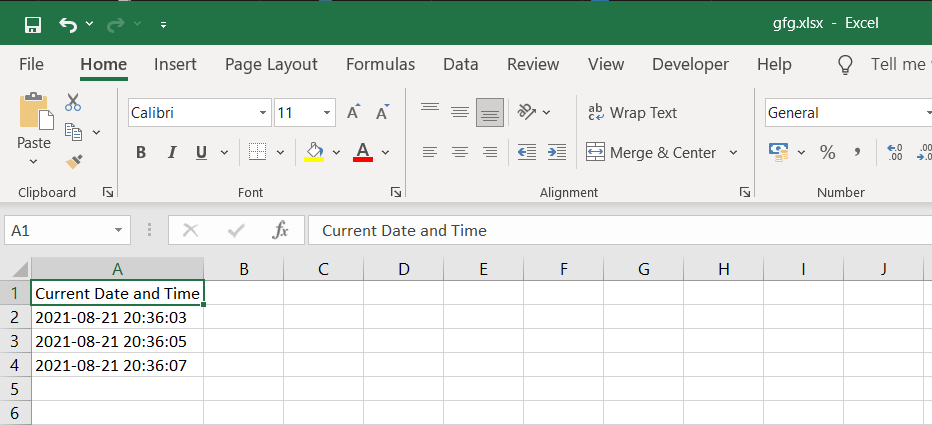How to add timestamp to excel file in Python

• Last Updated : 22 Nov, 2021

In this article, we will discuss how to add a timestamp to an excel file using Python.

Modules required

• datetime: This module helps us to work with dates and times in Python.
pip install datetime
• openpyxl: It is a Python library used for reading and writing Excel files.
pip install openpyxl
• time: This module provides various time-related functions.

Stepwise Implementation

Step 1: Create the workbook object and select the active sheet:

wb = Workbook()
ws = wb.active

Step 2 (Optional): Write the heading in cell A1.

# Here column=1 represents column A and row=1 represents first row.
ws.cell(row=1, column=1).value = "Current Date and Time"

Step 3: Use the following command to get the current DateTime from the system.

time = datetime.datetime.now().strftime('%Y-%m-%d %H:%M:%S')

The strftime() function is used to convert date and time objects to their string representation. It takes one or more inputs of formatted code and returns the string representation.

Step 4: Write the DateTime in cell A2.

ws.cell(row=2, column=1).value = time

Step 5: Initiate a sleep of 2 seconds using time.sleep(2)

Step 6: Similarly, get the current datetime again and write it in cell A3. Here you will notice a difference of 2 seconds from the previous DateTime since there was a sleep of 2 seconds in between.

Step 7: Finally, Save the excel workbook with a file name and close the workbook

wb.save('gfg.xlsx')
wb.close()

Below is the full implementation:

Python3

 # Import the required modulesimport datetimefrom openpyxl import Workbookimport time    # Main Functionif __name__ == '__main__':        # Create a workbook object    wb = Workbook()      # Select the active sheet    ws = wb.active      # Heading of Cell A1    ws.cell(row=1, column=1).value = "Current Date and Time"      # Cell A2 containing the Current Date and Time    ws.cell(row=2, column=1).value = datetime.datetime.now().strftime('%Y-%m-%d %H:%M:%S')      # Sleep of 2 seconds    time.sleep(2)      # Cell A3 containing the Current Date and Time    ws.cell(row=3, column=1).value = datetime.datetime.now().strftime('%Y-%m-%d %H:%M:%S')    time.sleep(2)      # Cell A4 containing the Current Date and Time    ws.cell(row=4, column=1).value = datetime.datetime.now().strftime('%Y-%m-%d %H:%M:%S')      # Save the workbook with a    # filename and close the object    wb.save('gfg.xlsx')    wb.close()

Output:My Personal Notes arrow_drop_up